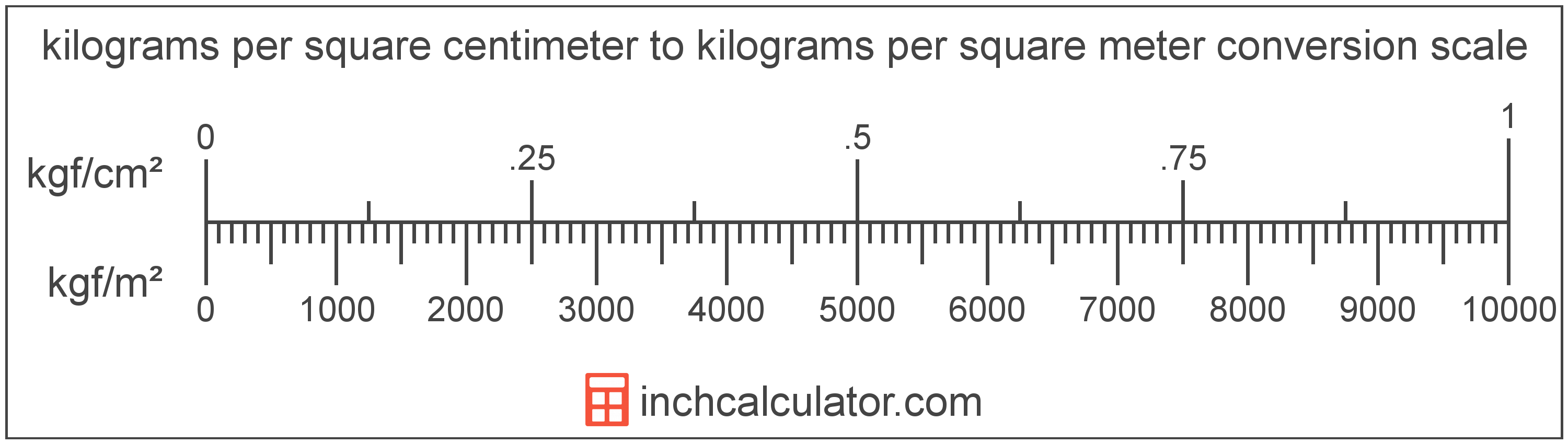# Kilograms per Square Meter to Kilograms per Square Centimeter Converter

Enter the pressure in kilograms per square meter below to get the value converted to kilograms per square centimeter.

Results in Kilograms per Square Centimeter:1 kgf/m² = 0.0001 kgf/cm²

## How to Convert Kilograms per Square Meter to Kilograms per Square Centimeter

To convert a measurement in kilograms per square meter to a measurement in kilograms per square centimeter, divide the pressure by the following conversion ratio: 10,000 kilograms per square meter/kilogram per square centimeter.

Since one kilogram per square centimeter is equal to 10,000 kilograms per square meter, you can use this simple formula to convert:

kilograms per square centimeter = kilograms per square meter ÷ 10,000

The pressure in kilograms per square centimeter is equal to the pressure in kilograms per square meter divided by 10,000.

For example, here's how to convert 5,000 kilograms per square meter to kilograms per square centimeter using the formula above.
kilograms per square centimeter = (5,000 kgf/m² ÷ 10,000) = 0.5 kgf/cm²Kilograms per square meter and kilograms per square centimeter are both units used to measure pressure. Keep reading to learn more about each unit of measure.

## What Are Kilograms per Square Meter?

One kilogram per square meter is the pressure of equal to one kilogram-force per square meter.

The kilogram per square meter is a non-SI metric unit for pressure. A kilogram per square meter is sometimes also referred to as a kilogram per square metre or kilogram-force per meter square. Kilograms per square meter can be abbreviated as kgf/m²; for example, 1 kilogram per square meter can be written as 1 kgf/m².

In the expressions of units, the slash, or solidus (/), is used to express a change in one or more units relative to a change in one or more other units. For example, kgf/m² is expressing a change in weight relative to a change in area.

The unit is deprecated and not permitted for use with SI units.

Kilograms per square meter can be expressed using the formula:
1 kgf/m2 = 1 kgf / m2

Pressure in kilograms per square meter are equal to the kilogram-force divided by the area in square meters.

## What Are Kilograms per Square Centimeter?

One kilogram per square centimeter is the pressure of equal to one kilogram-force per square centimeter.

The kilogram per square centimeter is a non-SI metric unit for pressure. A kilogram per square centimeter is sometimes also referred to as a kilogram per square centimetre or kilogram-force per centimeter square. Kilograms per square centimeter can be abbreviated as kgf/cm²; for example, 1 kilogram per square centimeter can be written as 1 kgf/cm².

The unit is deprecated and not permitted for use with SI units, although it is still in use as some precision measuring devices still use the unit.

Kilograms per square centimeter can be expressed using the formula:
1 kgf/cm2 = 1 kgf / cm2

Pressure in kilograms per square centimeter are equal to the kilogram-force divided by the area in square centimeters.

## Kilogram per Square Meter to Kilogram per Square Centimeter Conversion Table

Table showing various kilogram per square meter measurements converted to kilograms per square centimeter.
Kilograms Per Square Meter Kilograms Per Square Centimeter
1 kgf/m² 0.0001 kgf/cm²
2 kgf/m² 0.0002 kgf/cm²
3 kgf/m² 0.0003 kgf/cm²
4 kgf/m² 0.0004 kgf/cm²
5 kgf/m² 0.0005 kgf/cm²
6 kgf/m² 0.0006 kgf/cm²
7 kgf/m² 0.0007 kgf/cm²
8 kgf/m² 0.0008 kgf/cm²
9 kgf/m² 0.0009 kgf/cm²
10 kgf/m² 0.001 kgf/cm²
20 kgf/m² 0.002 kgf/cm²
30 kgf/m² 0.003 kgf/cm²
40 kgf/m² 0.004 kgf/cm²
50 kgf/m² 0.005 kgf/cm²
60 kgf/m² 0.006 kgf/cm²
70 kgf/m² 0.007 kgf/cm²
80 kgf/m² 0.008 kgf/cm²
90 kgf/m² 0.009 kgf/cm²
100 kgf/m² 0.01 kgf/cm²
200 kgf/m² 0.02 kgf/cm²
300 kgf/m² 0.03 kgf/cm²
400 kgf/m² 0.04 kgf/cm²
500 kgf/m² 0.05 kgf/cm²
600 kgf/m² 0.06 kgf/cm²
700 kgf/m² 0.07 kgf/cm²
800 kgf/m² 0.08 kgf/cm²
900 kgf/m² 0.09 kgf/cm²
1,000 kgf/m² 0.1 kgf/cm²
2,000 kgf/m² 0.2 kgf/cm²
3,000 kgf/m² 0.3 kgf/cm²
4,000 kgf/m² 0.4 kgf/cm²
5,000 kgf/m² 0.5 kgf/cm²
6,000 kgf/m² 0.6 kgf/cm²
7,000 kgf/m² 0.7 kgf/cm²
8,000 kgf/m² 0.8 kgf/cm²
9,000 kgf/m² 0.9 kgf/cm²
10,000 kgf/m² 1 kgf/cm²

## References

1. National Institute of Standards and Technology, NIST Guide to the SI, Chapter 6: Rules and Style Conventions for Printing and Using Units, https://www.nist.gov/pml/special-publication-811/nist-guide-si-chapter-6-rules-and-style-conventions-printing-and-using